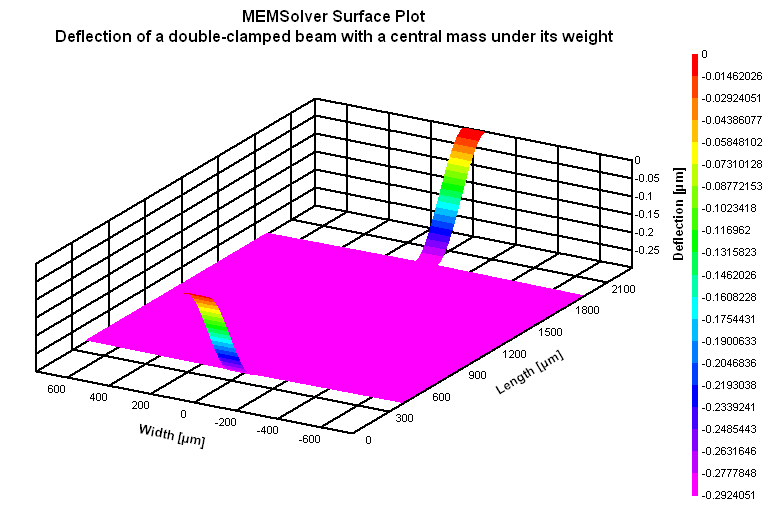CLPMASS,len,wid,thc,mlen,mwid,mthc,den,ymod,sel
Bending of a double-clamped beam with a central mass under its weight

len          length of beam in µm
wid         width of beam in µm
thc          thickness of beam in µm
mlen       length of the mass in µm
mwid      width of the mass in µm
mthc       thickness of the mass in µm
den         density of the material in kg/m3
ymod      Young's modulus of the material in GPa
sel          number denoting the selected result.
Use 1 for maximum stress, 2 for deflection and 3 for spring constantNotes

An inertial mass suspended from two or more clamped beams is a structure often used in MEMS accelerometers and other motion sensitive devices. A typical structure is as shown above. Due to the weight of the mass, the structure will have a displacement perpendicular to the surface in the unloaded condition.

Use this form to calculate the displacement of the mass due to its weight and the resulting stress in the beams. This displacement may generate an offset in the output of the sensor and should be considered in the analysis. If there are more springs supporting the mass connected in parallel, the effective spring constant will be the sum of spring constants of all the beams.

The X-Y plot gives the distribution of stress on the beam surface. It shows that the stress changes from positive maximum to negative maximum along the length of the beam. When the mass is pushed down, the surface stress near the clamped edge will be tensile and that near the mass will be compressive. he 2D and 3D surface plots shows the deflection of the beam mass system.Assumptions

-The default material is Silicon with a Young's modulus of 180GPa.
-The beam has uniform cross section.
-The weight of the beam and mass is uniformly distributed.
-There is no other load acting on the beam other than the weight of the mass and its own weight.
-The bending of the mass is negligible